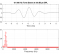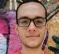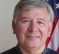## Measure for similarity of compressed/expanded signalsStarted by 6 years ago10 replieslatest reply 6 years ago122 views

Classic way to measure how 2 signals are similar is normalized correlation. I have not been been able to find a precise mathematical definition for similarity, but I assume s1 and s2 are defined similar  for correlation application if they are proportional, that is s1 = K.s2

My question is about similarity in a visual fashion, say s1 is a compressed or expanded version of s2. For example, s1 is a 100Hz sine wave and s2 is a 10Hz sine wave, with different amplitudes. These 2 are same signal(sine) but s1 is just s2 that is time stretched and scaled. Now extend this to a general signal. s2 is compressed version(not sure if this the right word, but you get the idea) of s1 if it undergoes the same amplitude variation, but in a shorter time, that is s1(t)=s2(a.t)

An illustration for this:

Time stretching

Then is there a method to signify this similarity?

[ - ]Check out https://en.wikipedia.org/wiki/Dynamic_time_warping for some ideas.  The basic idea is to allow the timing of one of the signals to be be locally "compressed" or "expanded" so that it best "matches" the other signal.  Then pick a traditional distance/similarity metric that you could use to quantify similarity between any two signals (e.g. correlation, root mean squared error, root median squared error).

[ - ]This is what I was looking for! Thanks a lot!

[ - ]By "visual fashion" I am going to assume you are talking about images.  If that is indeed the context, then I suggest the article "Image Quality Assessment: From Error Visibility to Structural Similarity", Wang, Z., Conrad Bovik, A., Rahim Sheikh, H., and Simoncelli, E. P., IEEE Transactions on Image Processing, Vol. 13, No. 4, April 2004.

Here is the abstract of the paper,

Objective methods for assessing perceptual image quality traditionally attempted to quantify the visibility of errors (differences) between a distorted image and a reference image using a variety of known properties of the human visual system.  Under the assumption that human visual perception is highly adapted for extracting structural information from a scene, we introduce an alternative complementary framework for quality assessment based on the degradation of structural information.  As a specific example of this concept, we develop a Structural Similarity Index and demonstrate its promise through a set of intuitive examples, as well as comparison to both subjective ratings and state-of-the-art objective methods on a database of images compressed with JPEG and JPEG2000.

[ - ]No my bad in wording maybe. I had 1-d signals in mind. By visual I meant we see two signals and can tell they are similar.

[ - ]Hi, aarash. Do you mean qualitative measures of similarity? It seems a bit vague to me what kind of comparison between s1 and s2 you intend to do (if it is not in the sense of Michael's answer). By "compressed version", does the words have the same meaning as in "get the coefs of s1 in some alternative domain (change the basis), cancel out the non significant coefs and transform back to time (and that is s2)"?

[ - ]No. I just found how to express it : s1(t)=K.s2(a.t)

We can assume k=1 because we want to normalize signal energies.

Edited original post.

An illustration for this:
Time stretching

[ - ]Well, OK, you have come up with an expression that relates two functions in an equality.  Rarely will that apply because there is noise.

A simple expansion using that idea would be:

s1(t)+n1(t) ~? K.s2(a.t)+n2(t)

where n2(t) could just as easily be K.n2(a.t).  But it *is* noise so maybe that's better and maybe it isn't.

Also, I've used ~? to indicate a comparision measure because that's really what you asked for.

I don't believe that proportionality is necessarily a good measure of similarity, particularly in the noisy cases.  Correlation is the best measure that I know of.  Without referring to texts on the subject, I'd simply say that:

Correlation of 1.0 is perfect "similarity".

Correlation of 0 is "no similarity"

Correlation of -1.0 is perfect "similarity" with opposite sign.

Perhaps a more compact way of saying this is:

Magnitude of correlation of 1.0 is "perfectly similar".

Magnitude of correlation of 0 is "perfectly dissimilar".

Magnitude of correlation between 0 and 1 is "somewhat similar".

But, in fact, using "similar" in place of "correlated" seems that one is adding an unnecessary term in our language.  I'd simply stick with "correlated" or "correlation coefficient".  Then one can add "under time compression / expansion" if need be.

Then one can imagine a 3-D surface of correlation coefficients with K and a as the x-y dimensions.  The peak of the surface (or peaks) indicate the best correlation.  The caution of doing something like this is illuminated by the following:

Take two noiseless sinusoids of different frequencies 1.0 and Pi BY DEFINITON.  Are they correlated? No.  Are they "similar" - that's a matter of definition.  I's rather say that they are correlated at a time compression factor of ....

[ - ]I understand that it's a good measure for general signals. But correlation method fails to use any additional information/restrictions(for example that signals might be time stretched). So it's not the best method for all applications.

[ - ]